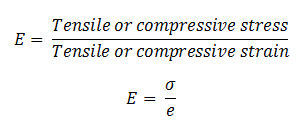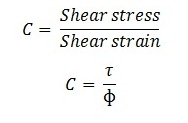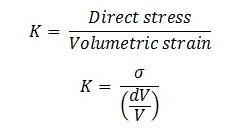# Elastic Constants – Young’s Modulus, Modulus of Rigidity and Bulk Modulus

In this article, you will learn about the Elastic Constant – Young’s modulus, Modulus of rigidity, and Bulk modulus. We will also discuss the difference between Young’s modulus, Modulus of rigidity and
Bulk modulus.The various elastic constants in the strength of materials are

1. Young’s modulus or modulus of elasticity
2. Modulus of rigidity or Shear Modulus
3. Bulk Modulus

Let’s discuss them one by one

### Young’s Modulus

Definition: It is defined as the ratio of tensile stress or compressive stress to the corresponding strain within elastic limit. It is denoted by symbol E. It is also known as modulus of elasticity or elastic modulus.Where

E = Young’s Modulus

σ = Tensile or compressive stress

e = Tensile or compressive strain

• The SI unit of Young’s modulus is N/mm2
.

### Modulus of Rigidity

Definition: It is defined as the ratio of shear stress to corresponding shear strain within elastic limit. It is also known as shear modulus. It is represented by C or G or N.Where

τ = Shear stress

ɸ = Shear strain

• The SI unit of C is N/mm2

### Bulk Modulus

Definition: It is defined as the ratio of direct stress to the corresponding volumetric strain within the tic limit. It is denoted by K.σ = Direct stress

(dV/V) = Volumetric strain

• The SI unit of C is N/mm2

### Difference Between Young’s Modulus, Modulus of Rigidity and Bulk Modulus.

 S.no Young’s Modulus Modulus of Rigidity Bulk Modulus 1. It is the ratio of tensile or compressive stress to the corresponding strain within elastic limit. It is the ratio of shear stress to the corresponding shear strain within elastic limit. It is the ratio of direct stress to the corresponding volumetric strain within elastic limit. 2. It is denoted by E. It is denoted by C or G or N. It is denoted by K. 3. E = stress/ strain C = shear stress/shear strain K = direct stress/ volumetric strain.

This is all about the Elastic constant – Young’s modulus, modulus of Rigidity,and Bulk modulus. And if you found this article informative and useful than don’t forget to like and share it.